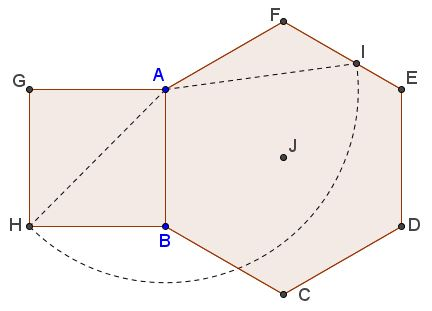# Golden Ratio in Hexagon

Tran Quang Hung has posted on the CutTheKnotMath facebook page a simple construction of the golden ratio in hexagon.

### Construction

Square $ABHG$ is constructed outside the hexagon $ABCDEF.$ Circle $(A, CH)$ with center at $A$ and radius $AH$ cuts $EF$ at $I$ in Golden Ratio:### Proof

Assume, without loss of generality, that $AB=AF=1.$ Then $AI=AH=\sqrt{2}.$

Set $\alpha = \angle FAI,$ $\beta =\angle AIF.$ Apply the Law of Sines in $\Delta AIF:$

$\displaystyle\frac{AF}{\sin\beta}=\frac{FI}{\sin\alpha}=\frac{AI}{\sin 120^{\circ}}.$

This can be rewritten as

$\displaystyle\frac{1}{\sin\beta}=\frac{FI}{\sin\alpha}=\frac{\sqrt{2}}{\sqrt{3}/2}=\frac{2\sqrt{6}}{3}.$

Thus we can identify $\beta:$ $\displaystyle\sin\beta=\frac{\sqrt{6}}{4}.$ Next we compute $\displaystyle\cos\beta=\frac{\sqrt{10}}{4}.$ Now, observe that $\alpha +\beta =60^{\circ},$ so that

\begin{align}\displaystyle \sin\alpha &= \sin (60^{\circ}-\beta)\\ &= \sin 60^{\circ}\cdot\cos\beta-\cos 60^{\circ}\cdot\sin\beta\\ &=\frac{\sqrt{3}}{2}\cdot\frac{\sqrt{10}}{4}-\frac{1}{2}\cdot\frac{2\sqrt{6}}{3}\\ &=\frac{\sqrt{6}}{8}(\sqrt{5}-1). \end{align}

From here

$\displaystyle FI = \sin\alpha\cdot\frac{2\sqrt{6}}{3}=\frac{\sqrt{6}}{8}(\sqrt{5}-1)\cdot\frac{2\sqrt{6}}{3}=\frac{\sqrt{5}-1}{2}=\frac{1}{\phi}.$

It follows that $\displaystyle\frac{EF}{FI}=\frac{FI}{EI}=\phi.$

A proof using Stewart's Theorem has been suggested by Leo Giugiuc. Apply Stewart's theorem to $\Delta AEF$ and the cevian $AI:$

$AF^{2}\cdot EI+AE^{2}\cdot FI=AI^{2}\cdot EF+EI\cdot FI\cdot AF.$

Substituting $AF=EF=1,$ $AI=\sqrt{2},$ $AE=\sqrt{3},$FI=x,EI=1-x$gives$(1-x)+3x=2+(1-x)x.$This reduces to the quadratic equation$x^{2}+x-1=0,$with the only positive solution$\displaystyle x=\frac{\sqrt{5}-1}{2},$which gives$1-x=\displaystyle\frac{3-\sqrt{5}}{2},$and, finally,$\displaystyle\frac{x}{1-x}=\phi.$### Remark Tran Quang Hung's construction that employs the diagonal of a square as a radius of a circle is akin to that of M. Bataille. Here's two constructions in the same diagram:According to M. Bataille,$\displaystyle\frac{BJ}{BK}=\phi;$according to Tran Quang Hung,$\displaystyle\frac{BL}{CL}=\frac{FI}{EI}=\phi.\$

A 2011 article by Quang Tuan Bui covers both cases and leads to a whole family of Golden Ratios in a regular hexagon. Tran Quang Hung's construction, like that of M. Battaille, has been discovered independently.### Golden Ratio